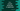# C++ program to convert lowercase to uppercase## C++ program to convert lowercase to uppercase:

In this post, we will learn how to convert a lowercase character to uppercase in C++. This program will take one character as input from the user and print the uppercase of that character.

This can be solved in different ways. Let’s try these one by one.

## Method 1: C++ program for lowercase to uppercase conversion by using ASCII values:

It is the easiest and most straightforward way to convert a lowercase to uppercase. The ASCII values of a to z ranges from 97 to 122. Similarly, the ASCII values of A to Z ranges from 65 to 92. The difference is 32 between a uppercase and lowercase character.

So, we can read the character and store it in a char variable and subtract 32 from it to get the uppercase value.

Complete program:

``````#include <iostream>
using namespace std;

int main()
{
char c;

cout << "Enter a lowercase character: " << endl;
cin >> c;
c -= 32;

cout << "It's uppercase character is: " << c << endl;

return 0;
}``````

In this program,

• c is a character variable.
• The program asks to enter a character, it reads that character and stores it in the variable c.
• Then, it subtracts 32 from c and stores that value in the same variable c.
• The last line is printing the uppercase character, i.e. c.

If you run this program, it will give output as like below:

``````Enter a lowercase character:
a
It's uppercase character is: A

Enter a lowercase character:
z
It's uppercase character is: Z``````

## Method 2: By using toupper() function:

toupper is a function defined in cctype header file. By using this function, we can convert a character to uppercase.

It is defined as like below:

``int toupper(int c)``

where, c is the character, casted to an integer.

It returns the ASCII value of the uppercase character. We can use this method to find out the uppercase of a given character as like below:

``````#include <iostream>
#include <cctype>

using namespace std;

int main()
{
char c;

cout << "Enter a lowercase character: " << endl;
cin >> c;
c = toupper(c);

cout << "It's uppercase character is: " << c << endl;

return 0;
}``````

This program is using toupper to convert c to uppercase and the result is stored in c.

If you run this program, it will give similar output.